1800-1023-196

+91-120-4616500

# Mass Fraction

## Relation between molality of solution (m) and mole fraction of the solute (XA).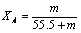Mass fraction: Mass fraction of a component in a solution is the mass of that component divided by the total mass of the solution. For a solution containing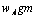of A and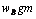of B

Mass fraction of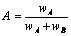; Mas fraction of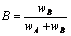It may be noted that molality, mole fraction, mass fraction etc. are preferred to molarity, normality, etc. because the former involve the weights of the solute and solvent where as later involve volumes of solutions. Temperature has no effect on weights but it has significant effect on volumes.

(10) Demal unit (D): The concentrations are also expressed in “Demal unit”. One demal unit represents one mole of solute present in one litre of solution at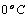.

## NEET & AIIMS Exam Sample Papers

 AIIMS SAMPLE PAPERS View More NEET SAMPLE PAPERS View More